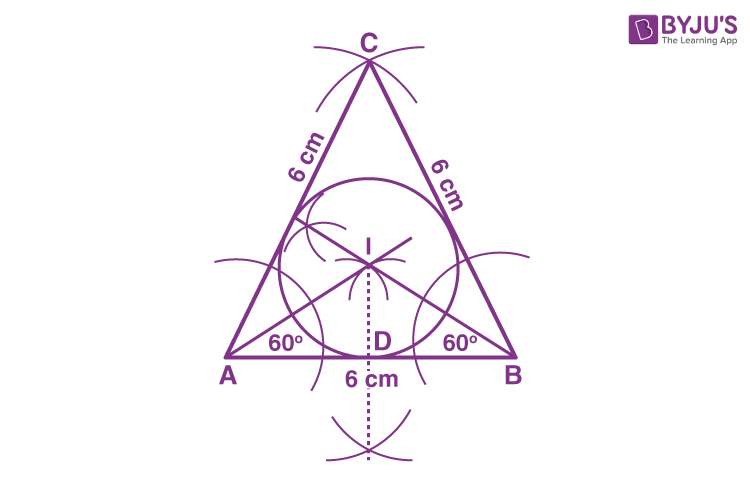# Construct an equilateral triangle of side 6 cm and mark the incircle.Step 1: Draw a line segment AB = 6cm.

Step 2: Construct an equilateral triangle by making an angle of 60° at A and B. The angles constructed will meet each other at point C.

Step 3:The angle bisectors are drawn for any two angles, say ∠A, ∠B

Step 4: The angle bisectors are extended to intersect each other at the point I which is the incentre of the circle.

Step 5: A perpendicular is drawn from I to any side of the triangle, say AB such that this perpendicular line meets the side at D .

Step 6: Using a compass, measure the distance DI and draw the circle which touches all three sides of the triangle.

Step 7: The obtained circle is the incircle of the given equilateral triangle.(1)(0)